###### Descriptions and Examples for the POV-Ray Raytracerby Friedrich A. Lohmüller Elementary Geometry for Raytracing
Italiano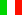Français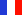Deutsch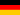Home
- POV-Ray Tutorial

- Geometrical Basics
for Raytracing

Right-angled Triangle
Pythagorean Theorem
Trigonometry Basics
Law of cosines
Equilateral Triangle
Regular Polygon
Polyhedron
Tetrahedron
Octahedron
Cube & Cuboid

Dodecahedron
Icosahedron
Cuboctahedron
Truncated Octahedron
Rhombicuboctahedron
Truncated Icosahedron
Circles
Tangent circles
Internal Tangents
External Tangents

- Geometric 3D Animations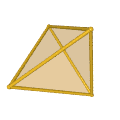## Regular TetrahedronSome useful geometrical facts

In the following we write for the square root of a number the expression "sqrt(ZAHL)"
conforming to the syntax used in POV-Ray.

 Dimensions Length of tetrahedron edge: a. The height of an regular tetrahedron: h = sqrt (2/3) * a ; The radius of circumsphere: R = sqrt( 3/8 ) * a ; The height of the incenter O or the radius of the insphere: r = 1/3 * R or   r = 1/sqrt(24) * a ; The radius of midsphere (tangent to edges): rm = 1/sqrt(8) * a ; The angle between two faces: ~ 70,53 (yellow) Angle(C,MAB,D) = degrees(atan(2*sqrt(2))); The angle between an edge and a face: ~ 54,74 (green) Angle(0,A,D) = degrees( atan(sqrt(2))); The angle vertex-center-vertex: ~ 109.471 (violet) Angle(A,0,D) = degrees( acos( -1/3 ));Two regular tetrahedron in a cubeA regular tetrahedron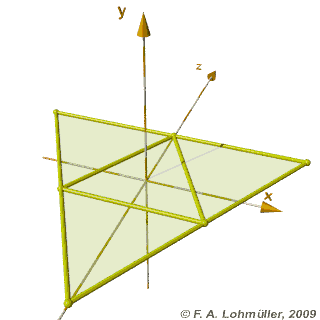Folding of a regular tetrahedronA tetrahedron of vectors
How to make it with POV-Ray
Animation in POV-RayMethan CH4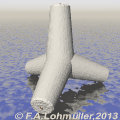Tetrapode
top

 © Friedrich A. Lohmüller, 2013 http://www.f-lohmueller.de